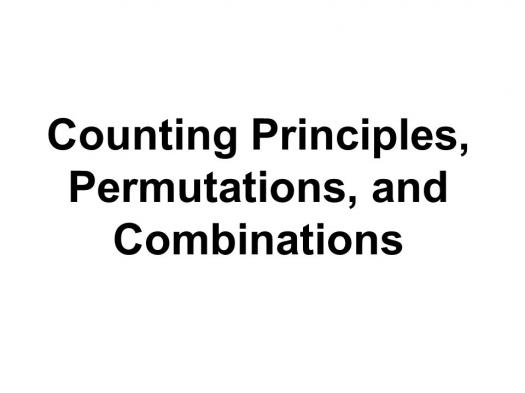# Counting, Permutations & Combinations Assessment Test

10 Questions | Total Attempts: 269SettingsTake this assessment test to assess your knowledge of counting principles, permutations, and combinations. Find out how to count the possible outcomes there are in various situations, factorial, permutations, combinations, and how to use them to find probabilities.

• 1.
An arrangement or ordering of a number of distinct objects is...
• A.

Permutation

• B.

Combination

• C.

Branching

• D.

Order

• 2.
Selections of some members of a set where order is disregarded is called...
• A.

Combination

• B.

Arrangement

• C.

Permutation

• D.

Nomination

• 3.
A permutation could be referred to as...
• A.

Nominal variable

• B.

Ordered combination

• C.

Lag variable

• D.

Outlier

• 4.
Which is an important parameter of permutation?
• A.

Number of items being chosen at a time

• B.

Only one item

• C.

Some items

• D.

Part of items

• 5.
Which is a type of permutation?
• A.

Cyclic

• B.

Round

• C.

Open

• D.

Real

• 6.
The product of all positive integers less than or equal to n is...
• A.

Figure

• B.

Number

• C.

N factorial

• D.

Factor

• 7.
How many different combinations of 3 numbers are there?
• A.

5

• B.

6

• C.

7

• D.

8

• 8.
How many different combinations of 4 items are there?
• A.

20

• B.

22

• C.

24

• D.

26

• 9.
How many combinations can be made with 5 numbers?
• A.

95

• B.

115

• C.

120

• D.

130

• 10.
How many different combinations of 8 numbers are there?
• A.

40320

• B.

40301

• C.

43202

• D.

40323

Related TopicsBack to top